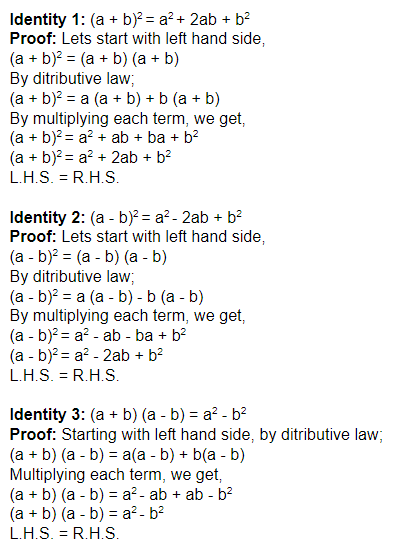Checkout JEE MAINS 2022 Question Paper Analysis : Checkout JEE MAINS 2022 Question Paper Analysis :

# Algebraic Identities For Class 8

Algebraic identities for class 8, will teach you the standard identities list, we use to solve the mathematical expressions, which are based on these formulas and identities. Also, students of the 8th standard will learn to prove these identities using distributive law and multiplications techniques.

As we know, identity is equality which is true for all values of the variable. These identities are the algebraic expressions, which defines that the Left-Hand Side(LHS) and the Right-Hand Side(RHS) of the equation is equal for all the values of the variable.

A variable is a term that can take any value. It can take any position in the number line, which has an infinite number of points. The value of algebraic expression changes with the changed value of the variable contained in it. The algebraic identities for class 8 also follow the same perception.

The algebraic expressions are usually expressed as monomials, binomials and trinomials based on one, two or three terms present in it. In fact, the expression which has one or more than one terms present in it is called a polynomial. The number attached to the term of an algebraic expression is called a coefficient.

The algebraic identities for class 8 consist of three major identities, which consist of algebraic expressions and is true for identity definition. The algebraic formulas for class 8 are also derived using these identities. These identities and formulas will be used to solve algebraic equations. Also, with the help of these identities, we can easily express any given equation which is relevant to the algebraic identities in the simpler form.

 Standard Algebraic Identities List (1) (a + b)2 = a2 + 2ab + b2 (2) (a – b)2 = a2 – 2ab + b2 (3) (a + b) (a – b) = a2 – b2

These are the general algebraic identities. If we put the values for a and b, in any of the above three expressions, the left-hand side of the equation will be equal to the right-hand side. Therefore, these expressions are called as identities.

Based on these identities, there are a number of algebraic formulas created. These formulas are used to solve algebraic problems. For class 8 and class 9 standard, these algebraic identities and formulas are commonly used. So, this article will be helpful for the students who are appearing for class 8 and class 9 exams.

As we already discussed algebraic identities, let us now discuss how to prove that these algebraic expressions are actually identities. These proofs will help you to solve many problems of algebraic questions for class 8 and class 9.

### Proof of Standard Algebraic IdentitiesHence, with this, all three identities are proved. Now let us solve some problems based on these identities.

## Algebra Identities Examples

Example 1: Solve (2x + 3) (2x – 3) using algebraic identities.

Solution: By the algebraic identity number 3, we can write the given expression as;

(2x + 3) (2x – 3) = (2x)2 – (3)2 = 4x2 – 9

Example 2: Solve (3x + 5)2 using algebraic identities.

Solution: We know, by algebraic identity number 1,we can write the given expression as;

(3x + 5)2 = (3x)2 + 2*3x*5 + 52

(3x + 5)2 = 9x2 + 30x + 25

Download BYJU’S – The Learning App and learn mathematical identities and formulas in an innovative and creative way.

 Related Links Algebraic Identities Chapter 9: Algebraic Expressions and Identities
Test your knowledge on Algebraic Identities For Class 8